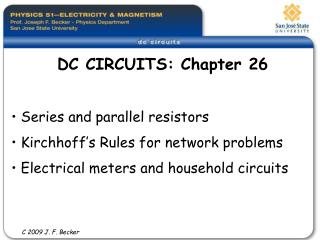DownloadDownload PresentationDC CIRCUITS: Chapter 26

# DC CIRCUITS: Chapter 26

Télécharger la présentation## DC CIRCUITS: Chapter 26

- - - - - - - - - - - - - - - - - - - - - - - - - - - E N D - - - - - - - - - - - - - - - - - - - - - - - - - - -
##### Presentation Transcript

1. DC CIRCUITS: Chapter 26 Series and parallel resistors Kirchhoff’s Rules for network problems Electrical meters and household circuits C 2009 J. F. Becker

2. DIRECT-CURRENT (DC) CIRCUITS In this chapter we will study methods of analyzing more complicated circuits having several sources, resistors, and other circuit elements. In general, we will find the current and power dissipation in each circuit element. First we consider ways to simplify resistors connected in a circuit in series and parallel.Second we consider Kirchhoff’s two rules to simplify more complicated networks. C 2009 J. F. Becker

3. Resistors connected in a circuit in series or parallel can be simplified using the following: Series connection Parallel connection

4. Method of simplifying the circuit in (a) below to get the equivalent resistance. We can then calculate the power P = I2 R dissipated in each resistor.

5. Ch. 26 #11: Four equivalent light bulbs R1 = R2 = R3 = R4 = 4.50 W, emf = 9.00 Volts.Find current and power in each light bulb. Which bulb is brightest? Later, if bulb #4 is removed which bulbs get brighter? Dimmer?

6. On course website, see old Test #1 from semesters S-04, F-03, and others for typical DC circuit problems, AND other typical problems on tests… C 2009 J. F. Becker

7. These complex circuits cannot be reduced to series – parallel combinations. So use Kirchhoff’s Rules: • S Ij = 0 (junction rule, valid at any junction); conservation of charge • S (DVj ) = 0 (loop rule, valid for any closed loop); conservation of energy

8. Use Kirchhoff’s junction rule at point “a” to reduce the number of unknown BRANCH currents from three totwo.

9. ELECTRICAL MEASURING INSTRUMENTS – METERSA d’Arsonval galvanometer meter movement:

10. AMMETERS have a very smallshunt resistor in them to reduce the effect of introducing the meter resistance into the circuit being measured.VOLTMETERS (DV) have a very largeseries resistor in them to reduce the amount of current drawn from the circuit being measured.

11. House wiring circuits

12. Hand drill circuit with ground wire for safety

13. Note: Section 26.4 Resistance-Capacitance (RC) Circuits will be deferred until later Next, back to the second half of Chapter 21: Chapter 21 Electric Field and Coulomb’s Law and Vectors Chapter 22 Gauss’s Law Chapter 23 Electric Potential Chapter 24 Capacitance and Dielectrics C 2009 J. F. Becker

14. Review See www.physics.edu/becker/physics51 C 2009 J. F. Becker